# Calculate v(t) and a of Functions Graph using Matlab xe), vC), a(t) 2ttt cox 2. lOt"-4t+2¢? t2(10t4t t3 1 +0 -...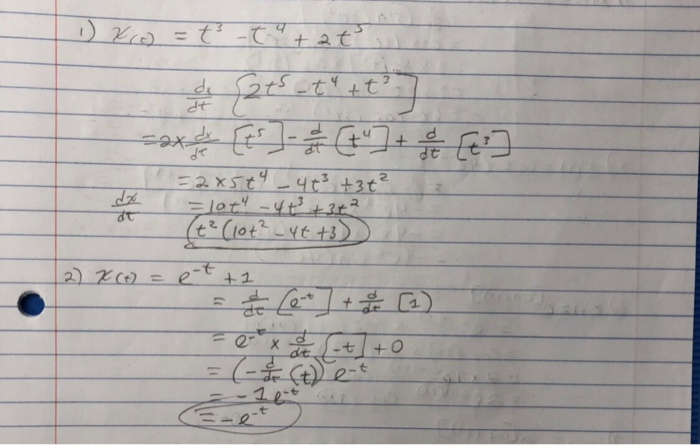Calculate v(t) and a of Functions Graph using Matlab xe), vC), a(t)
2ttt cox 2. lOt"-4t+2¢? t2(10t4t t3 1 +0 -t dt 1e -t

MATLAB CODE:

clc
clear all
close all

syms t

x(t) = t^3 - t^4 + 2*t^5; % Distance
v(t) = diff(x(t),t,1);    % Velocity
a(t) = diff(x(t),t,2);    % Acceleration

fprintf('x(t) = ')
disp(x(t))

fprintf('v(t) = ')
disp(v(t))

fprintf('a(t) = ')
disp(a(t))

subplot(3,1,1)
fplot(t,x(t),'k','LineWidth',2)
xlabel('t')
title('x(t)')
grid on

subplot(3,1,2)
fplot(t,v(t),'k','LineWidth',2)
xlabel('t')
title('v(t)')
grid on

subplot(3,1,3)
fplot(t,a(t),'k','LineWidth',2)
xlabel('t')
title('a(t)')
grid on

OUTPUT: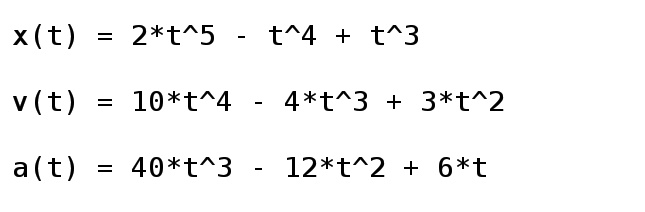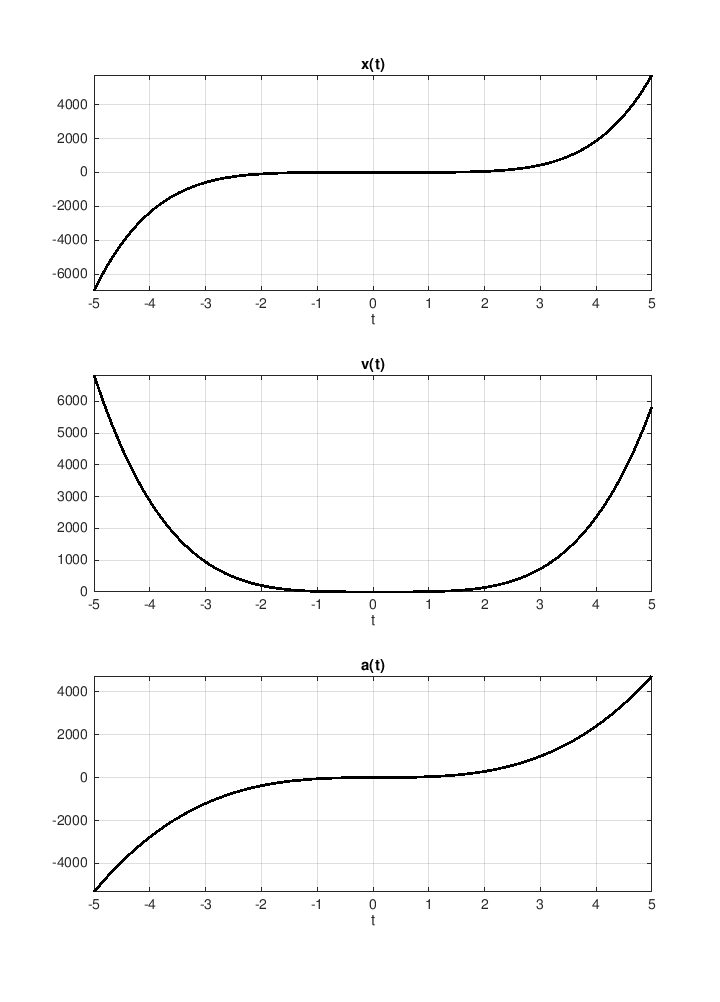##### Add Answer of: Calculate v(t) and a of Functions Graph using Matlab xe), vC), a(t) 2ttt cox 2. lOt"-4t+2¢? t2(10t4t t3 1 +0 -...
Similar Homework Help Questions
• ### using MATLAB solve for T3 if ALL other variable are known heat(1) == (m/M)*(((A-R)*(T3-T2))+((B/2)*(T3^2-T2^2))+((C/3)*(T3^3-T2^3))+((D/4)*(T3^4-T2^4))))

using MATLAB solve for T3 if ALL other variable are known heat(1) == (m/M)*(((A-R)*(T3-T2))+((B/2)*(T3^2-T2^2))+((C/3)*(T3^3-T2^3))+((D/4)*(T3^4-T2^4))))

• ### please solve problems 1 and problems 2. PROBLEM 1: Derive state-space equations for the following circuit in the form of L1 where χ = :L2 L3 L1 and (a) y 7 V L3 R1 L1 L3 R3 Vt R2 Vc し2 (c) For Part (...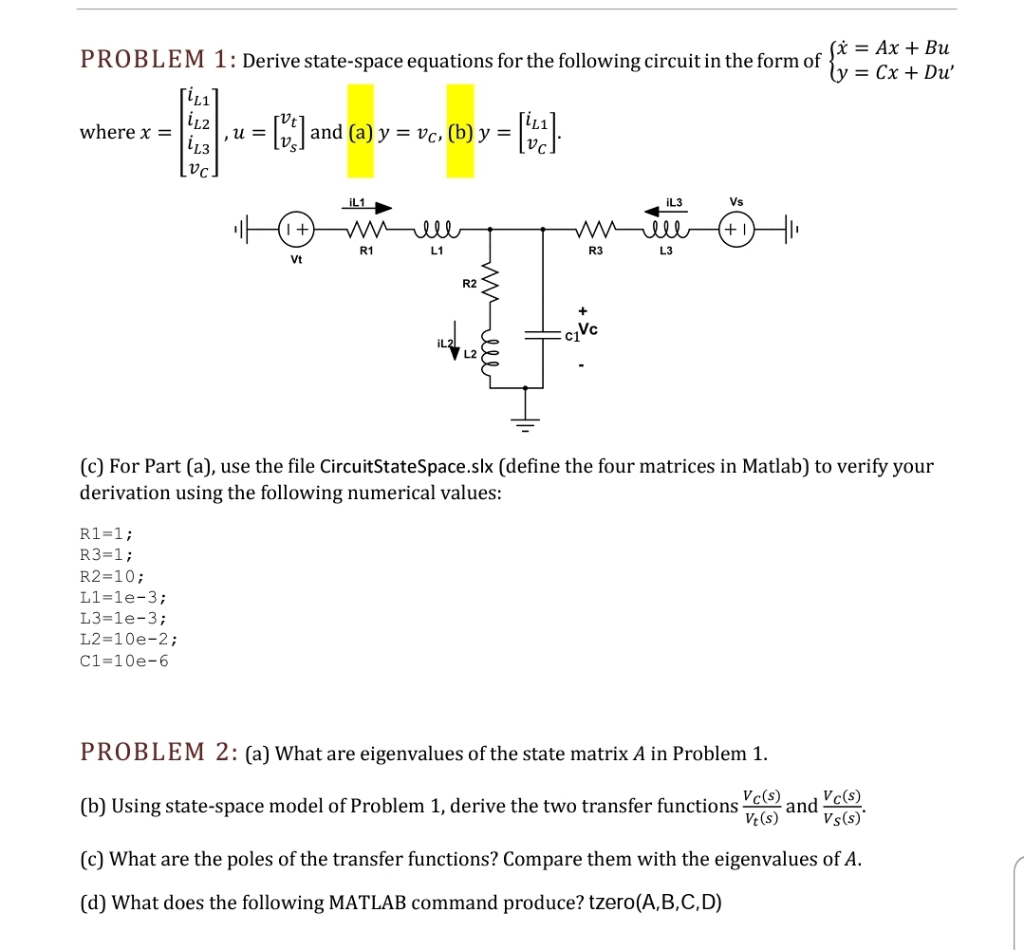please solve problems 1 and problems 2. PROBLEM 1: Derive state-space equations for the following circuit in the form of L1 where χ = :L2 L3 L1 and (a) y 7 V L3 R1 L1 L3 R3 Vt R2 Vc し2 (c) For Part (a), use the file CircuitStateSpace.slx (define the four matrices in Matlab) to verify your derivation using the following numerical values: R1-1; R3-1 R2-10; L1-1e-3 L3-1e-3 L2-10e-2 ; C1-10e-6 PROBLEM 2: (a) What are eigenvalues of the...

• ### 9. (a) Let Ao(x) = / (1-t*)dt, Ai(z) = / (1-t2) dt, and A2(z) = / (1-t2)dt. Compute these explici...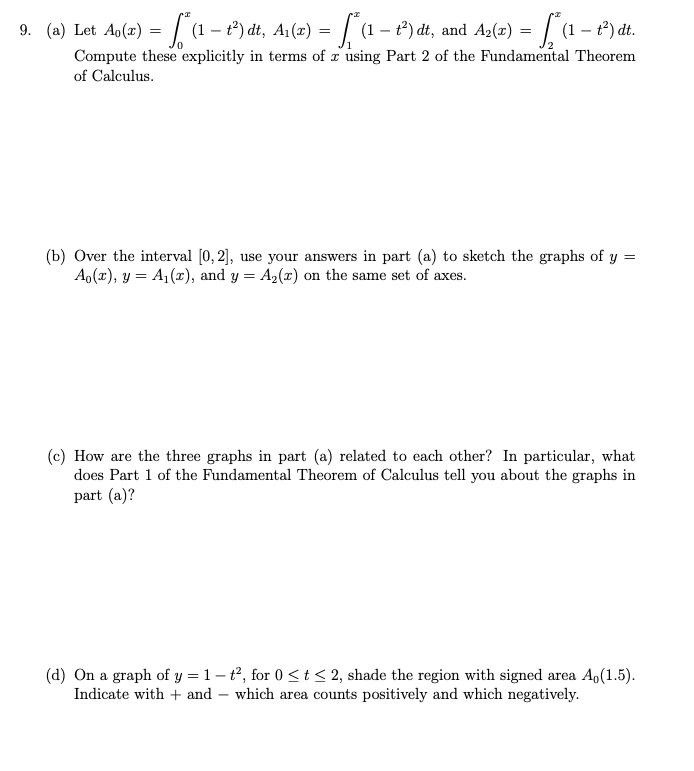9. (a) Let Ao(x) = / (1-t*)dt, Ai(z) = / (1-t2) dt, and A2(z) = / (1-t2)dt. Compute these explicitly in terms ofェusing Part 2 of the Fundamental Theorem of Calculus. b) Over the interval [0,2], use your answers in part (a) to sketch the graphs of y Ao(x), y A1(x), and y A2(x) on the same set of axes. (c) How are the three graphs in part (a) related to each other? In particular, what does Part 1 of...

• ### Matlab help 1) Given the functions x1()= tu()-tuft-1) and X2(t)=10e-5,11(), do the following: 1. Plotx,(t) and x2(0) using MATLAB 2. Use MATLAB to find and plot x(0=x:@*.x2(t), where * denotes co...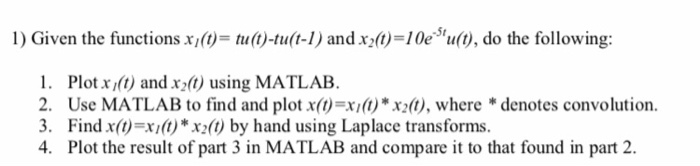Matlab help 1) Given the functions x1()= tu()-tuft-1) and X2(t)=10e-5,11(), do the following: 1. Plotx,(t) and x2(0) using MATLAB 2. Use MATLAB to find and plot x(0=x:@*.x2(t), where * denotes convolution. 3. Find x(t)=x;()*X2(1) by hand using Laplace transforms. 4. Plot the result of part 3 in MATLAB and compare it to that found in part 2. 2) Given the transfer function shown below, do the following: 1. Find the system's impulse response and plot it using MATLAB 2. Repeat...

• ### 2. (Chebyshev Polynomials). Below is a guideline for finding the coefficients in T,l(x) = cos(n cos-1 x), Chebyshev polynomials equivalently or T,,(cosa.) = cos(na). For example, To(x)-1, T1(x)=x, T2...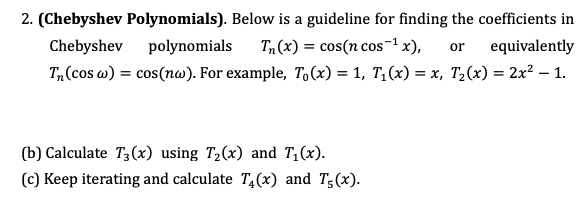2. (Chebyshev Polynomials). Below is a guideline for finding the coefficients in T,l(x) = cos(n cos-1 x), Chebyshev polynomials equivalently or T,,(cosa.) = cos(na). For example, To(x)-1, T1(x)=x, T2(x)= 2x2-1 (b) Calculate T3(x) using T2(x) and T1(x) (c) Keep iterating and calculate T(x) and T( 2. (Chebyshev Polynomials). Below is a guideline for finding the coefficients in T,l(x) = cos(n cos-1 x), Chebyshev polynomials equivalently or T,,(cosa.) = cos(na). For example, To(x)-1, T1(x)=x, T2(x)= 2x2-1 (b) Calculate T3(x) using T2(x)...

• ### 1.Solve the following problem over the interval from t 0 to 1 using a step size of 0.25 where y(0...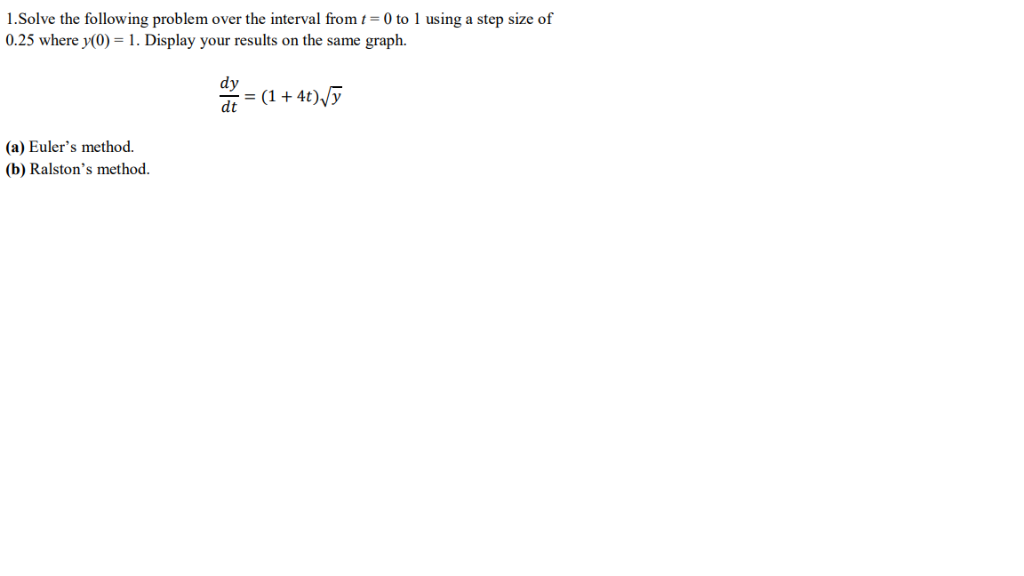1.Solve the following problem over the interval from t 0 to 1 using a step size of 0.25 where y(0) . Display your results on the same graph. dy dt (1 +4t)vy (a) Euler's method. (b) Ralston's method. 1.Solve the following problem over the interval from t 0 to 1 using a step size of 0.25 where y(0) . Display your results on the same graph. dy dt (1 +4t)vy (a) Euler's method. (b) Ralston's method.

• ### 2. For the transfer functions in problem 1 (a)(d)(e), find the corresponding impulse response functions h(t) using par...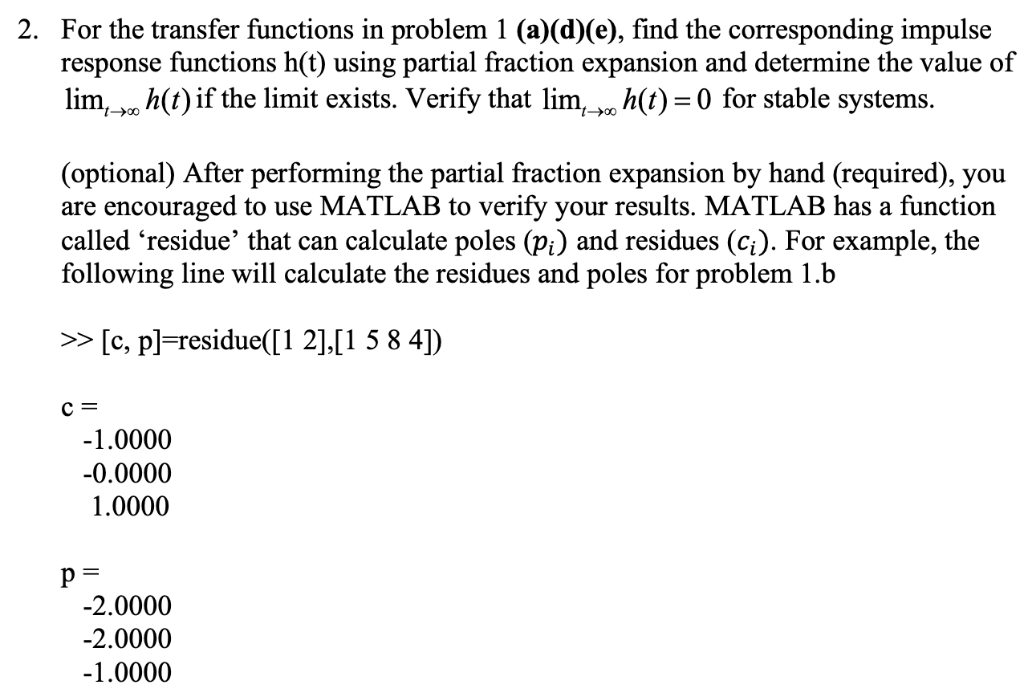2. For the transfer functions in problem 1 (a)(d)(e), find the corresponding impulse response functions h(t) using partial fraction expansion and determine the value of lim h(t) if the limit exists. Verify that lim- n(t)-0 for stable systems. (optional) After performing the partial fraction expansion by hand (required), yoiu are encouraged to use MATLAB to verify your results. MATLAB has a function called 'residue' that can calculate poles (pi) and residues (ci). For example, the following line will calculate the...

• ### 1. Write a short MATLAB script or function (m-file) that generates the functions (t)eu(t) for a =...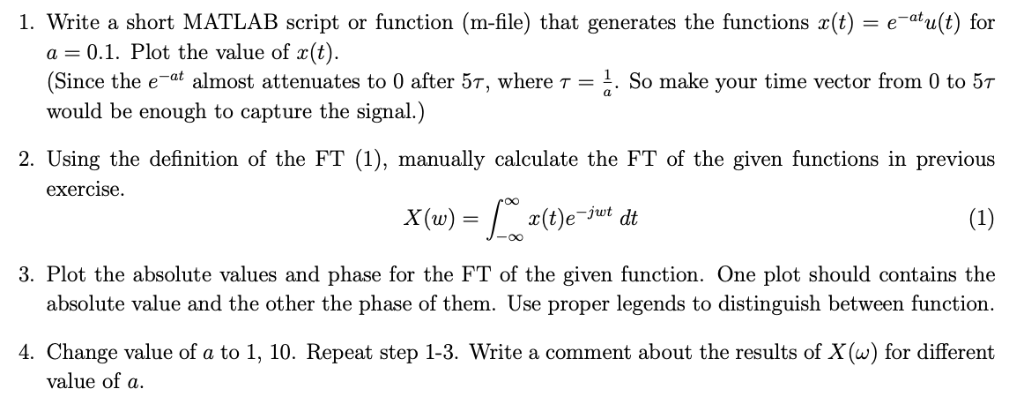Only calculate manually 1. Write a short MATLAB script or function (m-file) that generates the functions (t)eu(t) for a = 0.1. Plot the value of x(t). Since the e-af almost attenuates to 0 after 5T, where T1. So make your time vector from 0 to 5T would be enough to capture the signal.) 2. Using the definition of the FT (1), manually calculate the FT of the given functions in previous exercise 0O x(t)e 3. Plot the absolute values and...

• ### PLEASE SOLVE USING MATLAB As shown, two weights are in state of static equilibrium, hanging from three strings of known lengths. Write 6 equations and solve them in MATLAB to determine the angles (0,...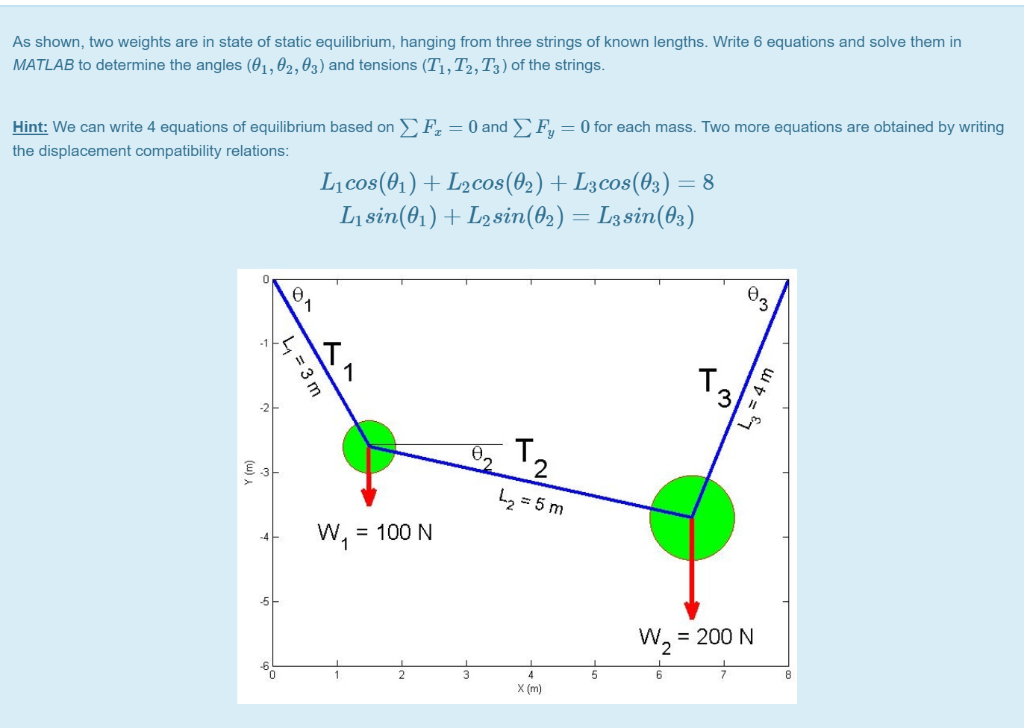PLEASE SOLVE USING MATLAB As shown, two weights are in state of static equilibrium, hanging from three strings of known lengths. Write 6 equations and solve them in MATLAB to determine the angles (0,, θ2,93) and tensions (T. T2, T3) of the strings. Hint: We can write 4 equations of equilibrium based on F 0and Fy- 0 for each mass. Two more equations are obtained by writing the displacement compatibility relations L1 cos(01)+L2cos(02) +Lacos(03) 8 L1 sin(θ 1 ) 0...

• ### Please write code in MATLAB. HW12_4: Solve the system of nonlinear equations over the interval 0 st0.03 using ode45. Display the results on the same graph. Include a legend. x(0)-3, y(0)-2, z(0)-1 ax...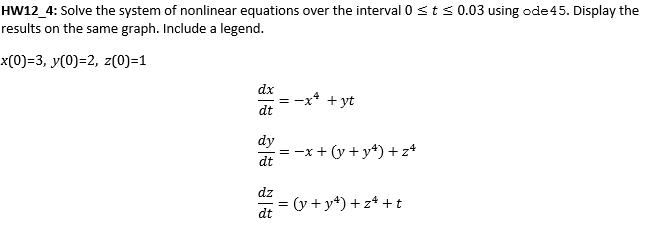Please write code in MATLAB. HW12_4: Solve the system of nonlinear equations over the interval 0 st0.03 using ode45. Display the results on the same graph. Include a legend. x(0)-3, y(0)-2, z(0)-1 ax dt dy dz HW12_4: Solve the system of nonlinear equations over the interval 0 st0.03 using ode45. Display the results on the same graph. Include a legend. x(0)-3, y(0)-2, z(0)-1 ax dt dy dz

Need Online Homework Help?